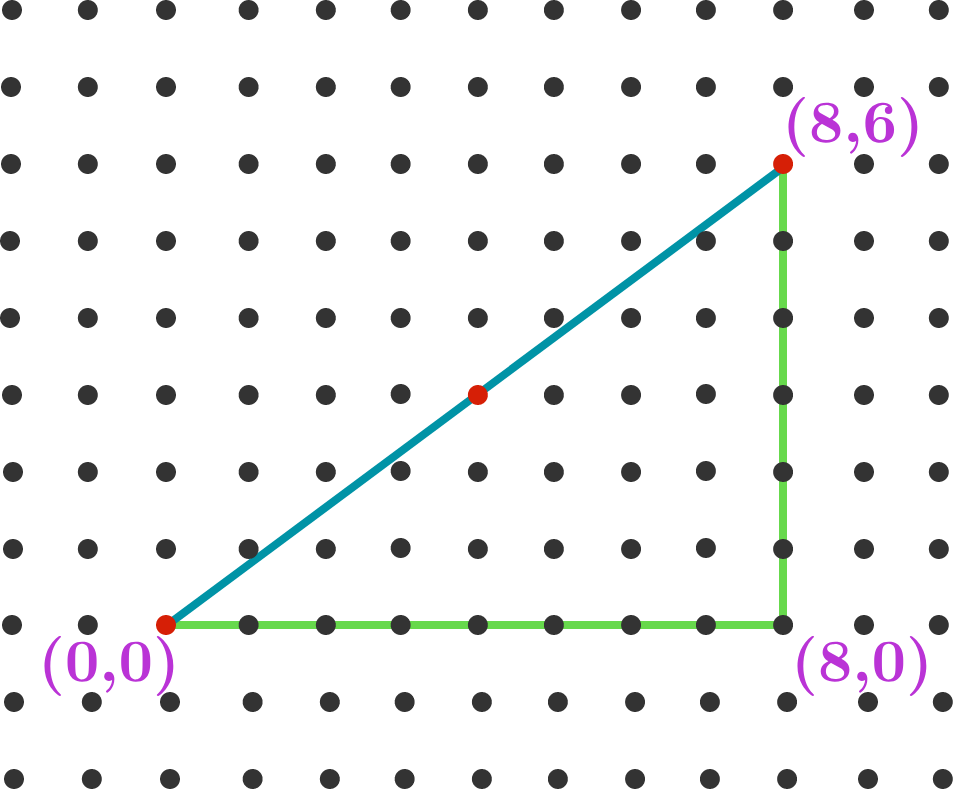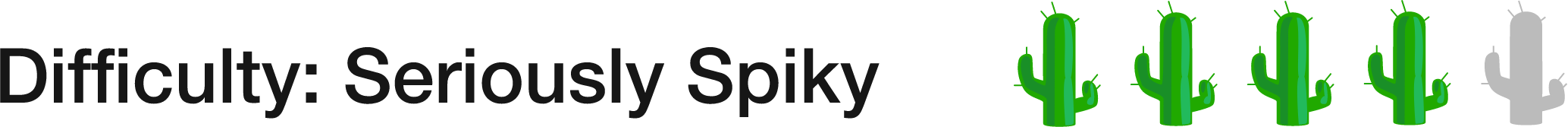# 14 of 100: Red Dot FeverIn the image above, 3 dots from the grid (marked red) lie on the hypotenuse of a right triangle. If the corners of the triangle were instead at $(0,0),$ $(1200,0),$ and $(1200,1000),$ how many dots from the grid would lie on the triangle's hypotenuse?If you don't know where to start, try hunting for a pattern with smaller examples!

×